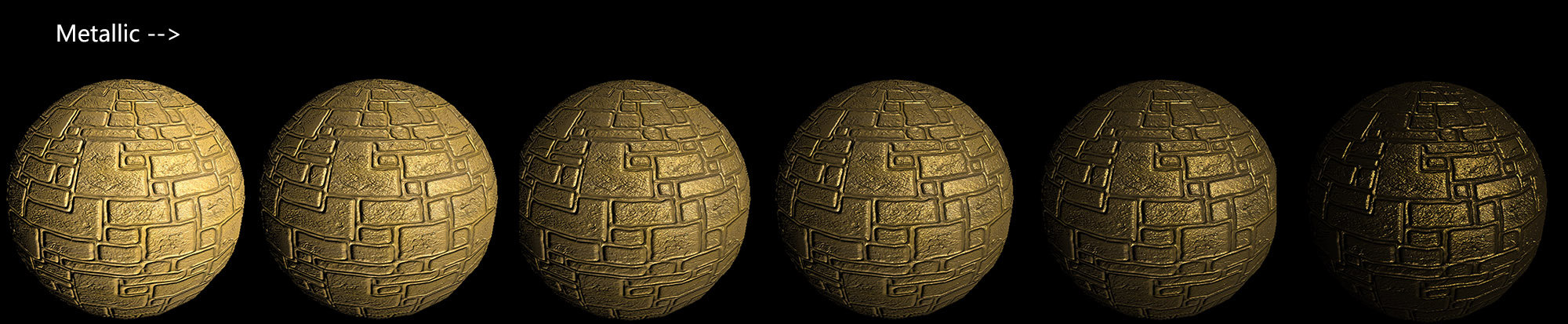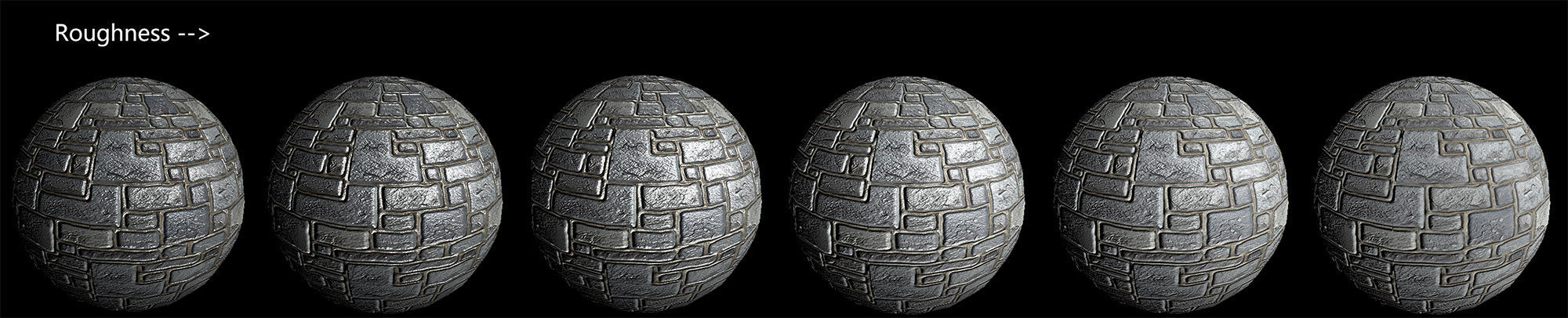# Jim's GameDev Blog# 实现基于物理的渲染

2016-6-5

struct appdata
{
float4 vertex : POSITION;
half2 texcoord : TEXCOORD0;
float3 normal : NORMAL;
float4 tangent : TANGENT;
};

struct v2f
{
float4 pos : SV_POSITION;
half2 uv : TEXCOORD0;
// 法线
float4 normal : TEXCOORD1;
// 切线
float4 tangent : TEXCOORD2;
// 副切线
float4 binormal : TEXCOORD3;
// 模型空间中的光源位置
float3 objLightPos : TEXCOORD4;
// 模型空间中的观察位置
float3 objViewPos : TEXCOORD5;
};

v2f vert(appdata i)
{
v2f o;

o.pos = mul(UNITY_MATRIX_MVP, i.vertex);
o.uv = TRANSFORM_TEX(i.texcoord, _MainTex);

float3 binormal = cross(i.normal, i.tangent.xyz) * i.tangent.w;
// 准备好 法线 切线 副切线
// w 分量存储模型空间中的顶点位置
o.normal = float4(i.normal, i.vertex.x);
o.tangent = float4(i.tangent.xyz, i.vertex.y);
o.binormal = float4(binormal, i.vertex.z);
// 模型空间中的光源位置
o.objLightPos = mul((float3x3)_World2Object, _worldLightPos).xyz;
// 模型空间中的观察位置
o.objViewPos = mul((float3x3)_World2Object, _WorldSpaceCameraPos).xyz;

return o;
}

half4 frag(v2f i) : SV_Target
{
// 切线空间矩阵
float3x3 obj2tan = float3x3(normalize(i.tangent.xyz), normalize(i.binormal.xyz), normalize(i.normal.xyz));
// 模型空间顶点位置
float3 objPos = float3(i.normal.w, i.tangent.w, i.binormal.w);
// 模型空间中光源位置
float3 objLightPos = i.objLightPos;
// 模型空间中光向量
float3 objLightDir = objLightPos - objPos;
// 模型空间中观察位置
float3 objViewPos = i.objViewPos;
// 模型空间中观察向量
float3 objViewDir = objViewPos - objPos;

// 切线空间光向量
float3 tanLightDir = normalize(mul(obj2tan, objLightDir));
// 切线空间观察向量
float3 tanViewDir = normalize(mul(obj2tan, objViewDir));
// 切线空间法线向量
float3 normaltex = tex2D(_BumpMap, i.uv) * 2 - 1;

half4 c = half4(1, 1, 1, 1);

// 在这里进行光照计算

return c;
}


$L_o(v) = \pi f(l_c,v) \otimes c_{light} \underline{ (n \cdot l_c) }$

$L_o(v)$ 是要求得的值，表示反射到眼中的光。

$f(l_c,v)$ 是 BRDF 方程，两个参数分别是入射光的反向向量和反射光向量。

$c_{light}$ 是入射光的辐照度。

$\underline{ (n \cdot l_c) }$ 是入射光的 Lambert 效应，如果入射光的反向向量和表面法线平行，那么这个值等于 1。下划线表示最小值为 0。

$\pi$ 来自于漫反射项。

$\otimes$ 表示各分量相乘。由于是使用 RGB 值来表示的，所以两个 RGB 值各通道值相乘。

c.rgb = PI * BRDF(tanViewDir, tanLightDir, normaltex) * _lightColor.rgb * _lightIntensity * max(dot (normaltex, tanLightDir), 0);


$BRDF = (1 - k) \cdot Diffuse + k \cdot Specular$

$BRDF = (1 - Metallic) \cdot Diffuse + Metallic \cdot Specular$

$f_{lambert \ diffuse} = { c_{diff} \over \pi }$

$c_{diff}$ 就是才是本身的固有色，也就是不受任何光照时的颜色。

Specular 项比较复杂，首先给出总的公式：

$f_{spec} = { F(l,h)G(l,v,h)D(h) \over 4(n \cdot l)(n \cdot v) }$

F 是 Fresnel 项，G 是微表面遮挡项，D 是微表面法线分布项，分母上表示的是一个矫正因子（一般会被 G 中某一部分抵消掉）。

$Fresnel_{Schlick} = c_{spec} + (1 - c_{spec})(1 - (l \cdot h))^5$

$c_{spec}$ 表示入射角为 $0^o$ 时的值，这是一个基础值，公式在此基础值只上模拟出近似 Fresnel 的曲线。至于这个值该如何选取可以参见参考资料中的一张关于 Fresnel 项的表格，其中详细记录了每一种材质对应的最理想的 $c_{spec}$ 值。一般在实现的时候会使用以下近似的方式来表示 $c_{spec}$，把他和 Metallic 值关联起来：

$c_{spec} = lerp(dielectricSpec, albedo, Metallic)$

dielectricSpec 是绝缘体的 $c_{spec}$ 值，albedo 是物体的固有色。

// 在这里进行光照计算
fixed4 albedo = tex2D(_MainTex, i.uv);

half3 diffColor = albedo * (1-_Metallic);
half3 diffTerm = DiffuseTerm(diffColor);

half3 specColor = lerp(_Specular.rgb, albedo, _Metallic.x);
half3 specTerm = SpecularTerm(specColor, _Roughness, tanViewDir, normaltex, tanLightDir);
c.rgb = PI * BRDF(tanViewDir, tanLightDir, normaltex) * _lightColor.rgb * _lightIntensity * max(dot (normaltex, tanLightDir), 0);

half3 DiffuseTerm(half3 diffColor)
{
return diffColor / Pi;
}

half3 SpecularTerm(half3 specColor, float roughness, half3 V, half3 N, half3 L)
{
half3 H = normalize(V + L);
half NdotL = saturate(dot(N, L));
half NdotV = saturate(dot(N, V));
half NdotH = saturate(dot(N, H));
half LdotH = saturate(dot(L, H));

half  D = Distribution_GGX(roughness, NdotH);
half  G = GeometricVisibility_Schlick(roughness, NdotV, NdotL);
half3 F = Fresnel_Schlick(specColor, LdotH);

return D * G * F / 4;
}

float Distribution_GGX(float roughness, float NdotH)
{
float m = roughness * roughness;
float m2 = m * m;
float d = (NdotH * m2 - NdotH) * NdotH + 1;
return m2 / (d*d);
}

float GeometricVisibility_Schlick(float roughness, float NdotV, float NdotL)
{
float k = roughness * roughness * 0.5;
float G_SchlickV = NdotV * (1 - k) + k;
float G_SchlickL = NdotL * (1 - k) + k;
return 0.25 / (G_SchlickV * G_SchlickL);
}

float3 Fresnel_Schlick(float3 specColor, float LdotH)
{
return specColor + (1 - specColor) * pow((1 - LdotH), 5);
}